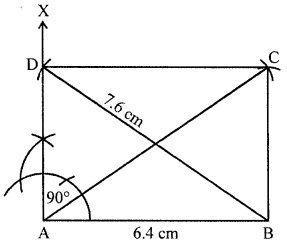# ML Aggarwal Class 8 Solutions for ICSE Maths Model Question Paper 5

## ML Aggarwal Class 8 Solutions for ICSE Maths Model Question Paper 5

Choose the correct answer from the given four options (1-2):
Question 1.
In a pentagonal pyramid number of faces, vertices and edges are
(a) F = 6, V = 6, E = 10
(b) F = 7, V = 7, E = 12
(c) F = 6, V = 8, E = 12
(d) none of these.
Solution:
In pentagonal pyramid
F = 6, V = 6, E = 10 (a)

Question 2.
If the lateral surface area of a cube is 144 cm , then its volume is
(a) 36cm3
(b) 216 cm3
(c) 288 cm3
(d) none of these
Solution:
Lateral surface area of a cube = 144 cm
∴ Edge = $$\sqrt{\frac{144}{4}}=\sqrt{36}$$ = 6 cm
and volume = (Edge)3 = (6)3 cm= 6 × 6 × 6 = 216 cm3 (b)

Question 3.
Draw the top, front and side views of the given solid.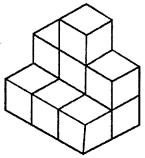Solution: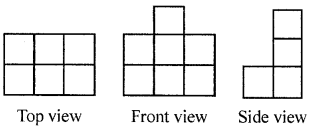Question 4.
The area of a trapezium is 96 cm2. If the lengths of its two parallel sides are 14 cm and 10 cm, then find the perpendicular distance between parallel sides.
Solution:
Area of a trapezium = 96 cm2
Lengths of two parallel sides are 14 cm and 10 cm
Then perpendicular distance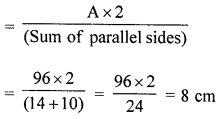Question 5.
In the given figure, C is the centre of the circle. The tangent to the circle of radius 6 cm from a point P outside the circle is of length 8 cm. Find the distance of the point P from the farthest point of the circumference.Solution:
C is the centre of the circle
Radius of the circle = 6 cm
and length of tangent PT = 8 cm
∵ CT is radius and PT is the tangent
∴ CT ⊥ PT at T Now in right ∆PTC
PC2 = PT2 + TC2 (Pythagoras Theorem)
= 82 + 62 = 64 + 36 = 100 = (10)2
∴ PC = 10 cm

Question 6.
Construct a quadrilateral PQRS in which PQ = 5.5 cm, RS = 6.5 cm, PS = 4.4 cm, PR = 6.5 cm and QS = 7.1 cm.
Solution:
Steps of construction :
(i) Draw a line segment SP = 4.4 cm.
(ii) With centre S and radius 6.5 cm and P with radius 6.5 cm,
draw arcs intersecting each other at R.
(iii) Join RS and RP.
(iv) Again with centre S and radius 7.1 cm and P with radius 5.5 cm
draw arcs intersecting each other at Q.
(v) Join QS and QP.
(vi) Join RQ also.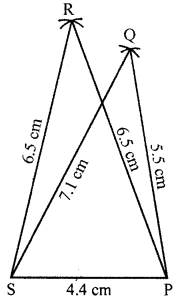Question 7.
The length, breadth and height of cuboidal water tank is 7 m, 6 m and 2 m respectively. If 4200 litre of water is pumped out from the tank. Find the fall in water level in the tank.
Solution:
Length of a cuboidal tank (l) = 7 m
and height (h) = 2 m
∴ Volume = Ibh = 7 × 6 × 2 = 84 m2
Capacity of water = 84 × 1000 litres = 84000 litres
4200 litres of water in pumped out
∴ $$\frac{4200}{1000}$$ = 4.2 m3 of volume is pumped out
∴ Level will fall = $$\frac{4.2}{l \times b}=\frac{4.2}{7 \times 6}=\frac{4.2}{42}$$
= $$\frac{1}{10}$$ m = 10 cm

Question 8.
Construct a rhombus PQRS such that PQ = 5.4 cm and ∠P = 60°. Also draw its lines of symmetry.
Solution:
Steps of construction :
(i) Draw a line segment PQ = 5.4 cm.
(ii) At P, draw a ray PX making an angle of 60°
and cut off PS = 5.4 cm.
(iii) With centre Q and S, and radius 5.4 cm,
draw arcs intersecting each other at R.(iv) Join QR and SR.
PQSR is the required rhombus.
Rhombus has 2 lines of symmetry.
The diagonals of the rhombus are the two line of symmetry.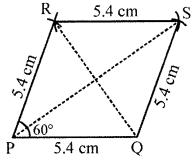Question 9.
A road roller has a diameter 84 cm and its width is 1.2 m. If it takes 500 complete revolutions to level a playground. Find the area of the playground. Also, find the cost of levelling the playground at a rate of 50 paise per m2.
Solution:
Diameter of roal roller = 84 cm
∴ Radius (r) = $$\frac{84}{2}$$ = 42 cm
Width (h)= 1.2 m = 120 cm
∴ Curved surface area = 2πrh
= 2 × $$\frac{22}{7}$$ × 42 × 120 cm2
= 31680 cm2
Area covered in 500 revolutions = 31680 × 500 cm2
= $$\frac{31680 \times 500}{100 \times 100}$$ m2 = 1584 m2
∴ Area of playground = 1584 m2

Question 10.
Construct a rectangle ABCD, such that AB = 6.4 cm and BD = 7.6 cm.
Solution:
Steps of construction :
(i) Draw a line segment AB = 6.4 cm.
(ii) At A draw a ray AX making an angle of 90°.
(iii) With centre B and radius 7.6 cm,
draw an arc which intersects AX at D.
(iv) Join BD.
(v) With centre A and radius 7.6 cm